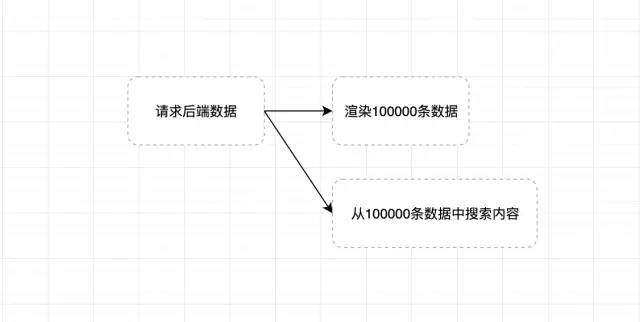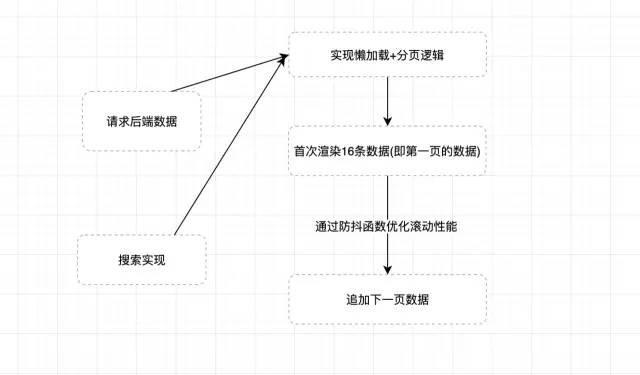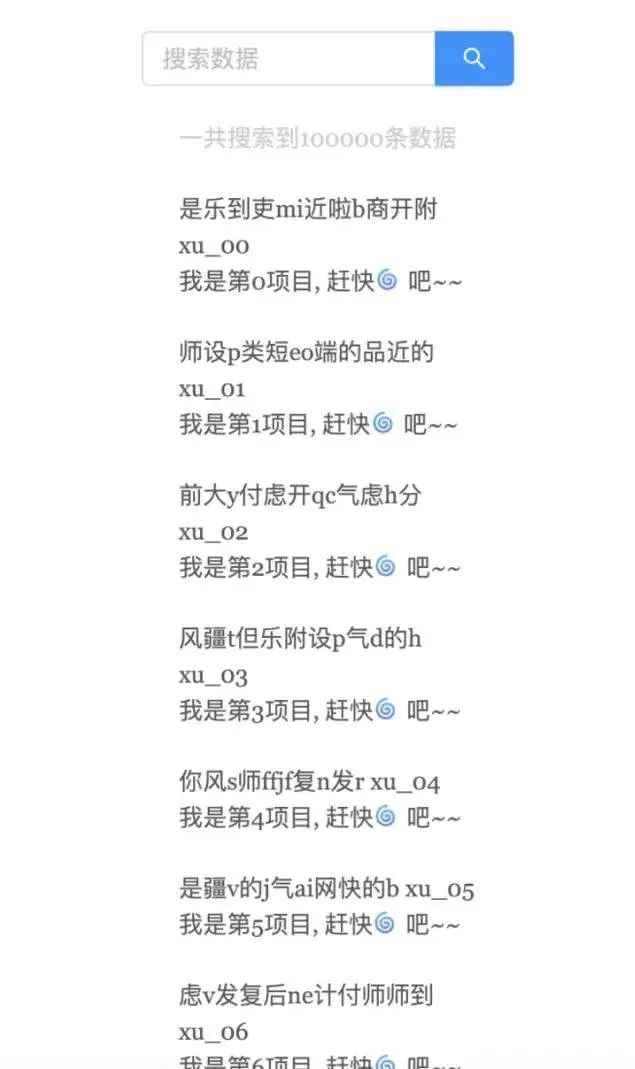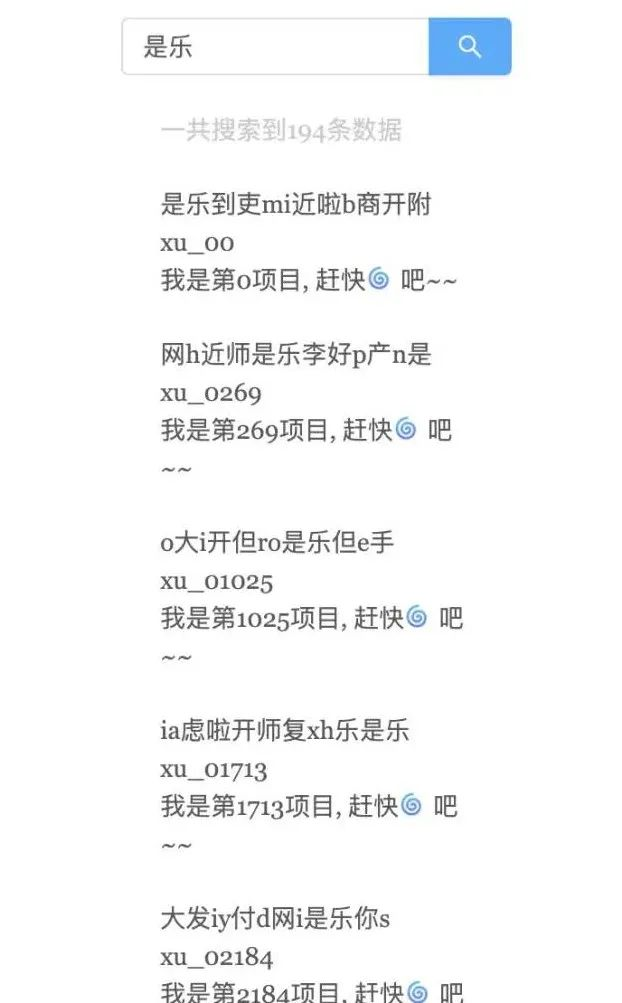# 前端工程师如何处理10万条数据

1. 采用懒加载+分页(前端维护懒加载的数据分发和分页)
2. 使用虚拟滚动技术(目前react的antd4.0已支持虚拟滚动的select长列表)

## 正文

```app.use(async (ctx, next) => {
if(ctx.url === '/api/getMock') {
let list = []

// 生成指定个数的随机字符串
function genrateRandomWords(n) {
let words = 'abcdefghijklmnopqrstuvwxyz你是好的嗯气短前端后端设计产品网但考虑到付款啦分手快乐的分类开发商的李开复封疆大吏师德师风吉林省附近',
len = words.length,
ret = ''
for(let i=0; i< n; i++) {
ret += words[Math.floor(Math.random() * len)]
}
return ret
}

// 生成10万条数据的list
for(let i = 0; i< 100000; i++) {
list.push({
name: `xu_0\${i}`,
title: genrateRandomWords(12),
text: `我是第\${i}项目, 赶快?吧~~`,
tid: `xx_\${i}`
})
}

ctx.body = {
state: 200,
data: list
}
}
await next()
})```

### 初级工程师的方案1. 请求后端数据:
```fetch(`\${SERVER_URL}/api/getMock`).then(res => res.json()).then(res => {
if(res.state) {
data = res.data
setList(data)
}
})```
1. 渲染页面
```{
list.map((item, i) => {
return <div className={styles.item} key={item.tid}>
<div className={styles.tit}>{item.title} <span className={styles.label}>{item.name}</span></div>
<div>{item.text}</div>
</div>
})
}```
1. 搜索数据
```const handleSearch = (v) => {
let searchData = data.filter((item, i) => {
return item.title.indexOf(v) > -1
})
setList(searchData)
}```

### 中级工程师的方案1. 懒加载+分页方案 懒加载的实现主要是通过监听窗口的滚动, 当某一个占位元素可见之后去加载下一个数据,原理如下:
这里我们通过监听window的scroll事件以及对poll元素使用getBoundingClientRect来获取poll元素相对于可视窗口的距离, 从而自己实现一个懒加载方案.

```function scrollAndLoading() {
if(window.scrollY > prevY) { // 判断用户是否向下滚动
prevY = window.scrollY
if(poll.current.getBoundingClientRect().top <= window.innerHeight) {
// 请求下一页数据
}
}
}

useEffect(() => {
// something code
return () => {
window.removeEventListener('scroll', getData, false)
}
}, [])```

• curPage当前的页数
• pageSize 每一页展示的数量
• data 传入的数据量

```let data = [];
let curPage = 1;
let pageSize = 16;
let prevY = 0;

// other code...

if(window.scrollY > prevY) { // 判断用户是否向下滚动
prevY = window.scrollY
if(poll.current.getBoundingClientRect().top <= window.innerHeight) {
curPage++
setList(searchData.slice(0, pageSize * curPage))
}
}
}```
1. 防抖函数实现 防抖函数因为比较简单, 这里直接上一个简单的防抖函数代码:
```function debounce(fn, time) {
return function(args) {
let that = this
clearTimeout(fn.tid)
fn.tid = setTimeout(() => {
fn.call(that, args)
}, time);
}
}```
1. 搜索实现 搜索功能代码如下:
```const handleSearch = (v) => {
curPage = 1;
prevY = 0;
searchData = data.filter((item, i) => {
// 采用正则来做匹配, 后期支持前端模糊搜索
let reg = new RegExp(v, 'gi')
return reg.test(item.title)
})
setList(searchData.slice(0, pageSize * curPage))
}```### 高级工程师的方案

```function multistep(steps,args,callback){

setTimeout(function(){